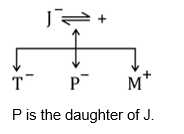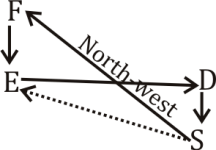# Reasoning Quiz (Data sufficiency) for SBI PO PRE 2019| 25th January 2019

Reasoning Questions and Reasoning Quiz for SBI exam Reasoning, IBPS PO Reasoning , IBPS Clerk Reasoning , IBPS RRB Reasoning , , and other competitive exams.

Directions (Q1 – Q5): Each of the questions below consists of a question and two statements numbered I and II given below it. You have to decide whether the data provided in the statements are sufficient to answer the question. Read both the statements and give answer.

(a) if the data in statement I alone are sufficient to answer the question, while the data in statement II alone are not sufficient to answer the question.

(b) if the data in statement II alone are sufficient to answer the question, while the data in statement I alone are not sufficient to answer the question.

(c) if the data either in statement I alone or in statement II alone are sufficient to answer the question.

(d) if the data even in both the statements I and II together not sufficient to answer the question.

(e) if the data in both the statements I and II together are necessary to answer the question.

Q1. How many students are there between Suresh and Mohan in a row of 50 students ?

I. Suresh is twelfth from the left end and Mohan is seventeenth from the right end.

II. Suresh is six places away from Jayesh, who is twentieth from the left end.

Q2. What does ‘\$’ mean in a code language?

I.‘5 \$ # 3’ means ‘flowers are really good’.

II.‘7 # 3 5’ means ‘good flowers are available’.

Q3. How is P related to J?

I. M is the brother of P and T is the sister of P.

II. P’s mother is married to J’s husband, who has one son and two daughters.

Q4. How is ‘never’ written in a code language ?

I. ‘never ever go there’ is written as ‘na ja ni ho’ in that code language.

II.‘go there and come back’ is written as ‘ma ho sa ni da’in that code language.

Q5. Among M, P, K, J, T and W, who is lighter than only the heaviest?

I. P is heavier than M and T.

II. W is heavier than P but lighter than J, who is not the heaviest.

Directions (6 to 10): Each of the following questions below consists of a question and two statements numbered I and II given below it. You have to decide whether the data provided in the statements are sufficient to answer the question. Read both the statements and give answer.

(a) If the data in statement I alone are sufficient to answer the question, while the data in statement II alone are not sufficient in answer the question.

(b) If the data in statement II alone are sufficient to answer the question, while the data in statement I alone are not sufficient to answer the question.

(c) If the data in either in statement I alone or in statement II alone are sufficient to answer the question.

(d) If the data in both the statements I and II together are not sufficient to answer the question.

(e) If the data in both the statements I and II are together necessary to answer the question.

6. Who amongst P, Q, R, S and T is the tallest?

I. P is taller than Q. T is not the tallest.

II. R is taller than P. S is not the tallest.

7. In which direction is point E, with reference to point S?

I. Point D is to east of point E. Point E is to the south of point F.

II. Point F is to the north-west to point S. Point D is to the north of point S.

8. In which month of the year did Raman go abroad for a vacation?

I. Raman correctly remembers that he went for a vacation in the first half of the year.

II. Raman’s son correctly remembers that they went for a vacation after 31st March but before 1st May.

9. On which day of the same week is Rudra’s exam scheduled (Monday being the first day of the week)?

I. Rudra correctly remembers that his exam is scheduled on a day after Tuesday, but before Thursday of the same week.

II. Rudra’s father correctly remembers that Rudra’s exam is scheduled on the third day of the week.

10. How may marks has Sujit scored in the test?

I. Sujit scored two-digit marks. His marks were less than or equal to 20.

II. Sujit scored more than 9 marks in the test.

# Solution

Q1. Ans.(a)

From statement I:

11 → Suresh 4 ← 21 →  Mohan← 16

So, there are 21 students between Suresh and Mohan.

Using statement II:

Mohan’s name is not even mentioned in the given statement. So, we cannot find the answer using statement II alone.

Q2. Ans.(e)

Using either of the statements alone we cannot find the code for \$, but using both the statements together we can find that ‘#53′ is ‘good flowers are’. So, the code for \$ is ‘really’.

Q3. Ans.(e)

Using statement, I:

We cannot find the relation using this statement as J’s name is not even mentioned in it.

Using statement II:We can say that J is the mother of P but cannot decide whether P is the son or daughter of J.

Using both statements together:Q4. Ans.(d)

Using either of the statements alone we cannot find the code, and even by using both the statements together we can only find that ‘never ever’ is coded as ‘naja’ the code for ‘never’ cannot be uniquely determined even by using both the statements together.

Q5. Ans.(e)

Using either of the statements alone we cannot find the answer, but when we use both the statements together we can find the relation in terms of weight, ie

K>J>W>P>M,T

6.(e)

From I: P> Q, but T is not the tallest.

From II: R> P, but S is not the tallest.

From I and II: R > P > Q. Neither S nor T can be the tallest. Hence R is the tallest.

1. (e)

From II:From I and II:Point E is to the north-west of Point S.

1. (b)

From I: Possible months: January, February, March, April, May or June.

From II: Raman’s son correctly remembers that his father went on vacation after 31st March but before 1st May.

So his father went on vacation in the month of April.

Hence only II is sufficient.

1. (c)

Sol. From I: The possible day of exam is Wednesday.

From II: The third day of the week is Wednesday.

Hence, either statement I alone or statement II alone is sufficient.

1. (d)

From I: Two-digit marks is less than or equal to 20.

Possible marks: 10, 11, 12, 13, 14, 15, 16, 17, 18, 19 and 20.

From II:Sujit scored more than 9 marks.

Possible marks: 10, 11, 12, 13, 14, 15, 16, 17, 18, 19 and 20.

Hence, statement I and II together are not sufficient.

## WhatsApp Group Join here

Mail us at : ambitiousbaba1@gmail.com